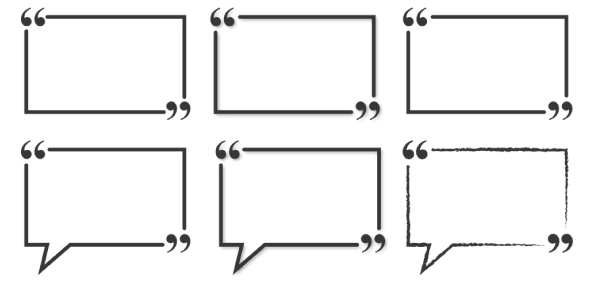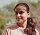Which of these cannot be the largest shape? I have different shapes. - ProProfs DiscussTopicsMoreProductsMore+ Ask Question

# Which of these cannot be the largest shape?Change Image    Delete

I have different shapes. The triangle is smaller than the rectangle. The rectangle is larger than the circle. The circle is smaller than the square. The octagon is larger than the triangle. The pentagon is smaller than the octagon. The ellipse is larger than the pentagon.

A. Rectangle
B. Circle
C. Octagon
D. Square
E. None of these

This question is part of SA Interns 2012 Recruitment
Asked by Signalalliance, Last updated: Jul 01, 2020

###Request 0FollowShareAnswer AnonymouslyAnswer LaterCopy Link#### J. Emmerich

J. Emmerich, Web Content Writer, New York City

In order to solve this problem, it is necessary to think about all of the given details. It was mentioned that the triangle is smaller than the rectangle. The circle is also smaller than the rectangle. The circle is also smaller than the square. The octagon is larger than the triangle which was mentioned earlier is smaller than the rectangle.

The Pentagon is smaller than the octagon which is also larger than the triangle. The ellipse is also larger than the pentagon. From the given details, all of the other choices are mentioned to be larger than the other shapes which means that the answer is B. Circle as it is the only shape that is mentioned to be smaller than the others.#### Anika Nicole

Content Writer, Teacher

Anika Nicole, Wordsmith, PG In Journalism, New York

To solve this problem, I gave a particular name to all the shapes which are mentioned in the question.

"T" for the triangle.

"R" for the rectangle.

"C" for the circle.

"S" for the square.

"O" for the octagon.

"P" for the polygon.

"E" for the ellipse.

After this, I created equations on the given conditions in question itself.

T is less than R.

R is greater than C.

C is less than S.

O is greater than T.

P is less thanO.

E is greater than P.

After considering all these six equations, I got a conclusion, that Circle cannot be the largest out of the given 7 shapes. Octagonal and Ellipse might be bigger. Hence, the correct answer is Circle.#### John Smith

John SmithCircleSearch for Google imagesSelect a recommended image
Upload from your computerCancelSearch for Google imagesSelect a recommended image
Upload from your computerCancelSearch for Google imagesSelect a recommended image
Upload from your computerCancel